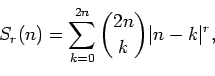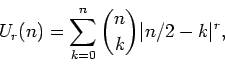Journal of Integer Sequences, Vol. 18 (2015), Article 15.3.2

## Generalizing Tuenter’s Binomial Sums

### Richard P. Brent Mathematical Sciences Institute Australian National University Canberra, ACT 2614 Australia

Abstract:

Tuenter considered centered binomial sums of the formwhere r and n are non-negative integers. We consider sums of the formwhich are a generalization of Tuenter's sums and may be interpreted as moments of a symmetric Bernoulli random walk with n steps. The form of Ur(n) depends on the parities of both r and n. In fact, Ur(n) is the product of a polynomial (depending on the parities of r and n) times a power of two or a binomial coefficient. In all cases the polynomials can be expressed in terms of Dumont-Foata polynomials. We give recurrence relations, generating functions and explicit formulas for the functions Ur(n) and/or the associated polynomials.

Full version:  pdf,    dvi,    ps,    latex

(Concerned with sequences A000142 A000364 A001147 A001469 A001813 A002105 A009843 A036970 A047053 A054879 A083061 A160485 A245244.)

Received July 16 2014; revised versions received January 18 2015; January 25 2015. Published in Journal of Integer Sequences, January 26 2015.